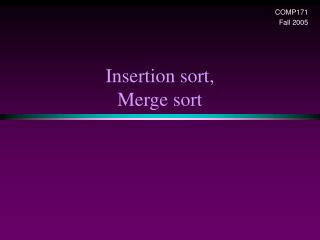DownloadDownload PresentationInsertion sort, Merge sort

# Insertion sort, Merge sort

Download Presentation## Insertion sort, Merge sort

- - - - - - - - - - - - - - - - - - - - - - - - - - - E N D - - - - - - - - - - - - - - - - - - - - - - - - - - -
##### Presentation Transcript

1. COMP171 Fall 2005 Insertion sort,Merge sort

2. Insertion sort 1) Initially p = 1 2) Let the first p elements be sorted. 3) Insert the (p+1)th element properly in the list so that now p+1 elements are sorted. 4) increment p and go to step (3)

3. Insertion Sort

4. Insertion Sort... see applet http://www.cis.upenn.edu/~matuszek/cse121-2003/Applets/Chap03/Insertion/InsertSort.html • Consists of N - 1 passes • For pass p = 1 through N - 1, ensures that the elements in positions 0 through p are in sorted order • elements in positions 0 through p - 1 are already sorted • move the element in position p left until its correct place is found among the first p + 1 elements

5. Extended Example To sort the following numbers in increasing order: 34 8 64 51 32 21 P = 1; Look at first element only, no change. P = 2; tmp = 8; 34 > tmp, so second element is set to 34. We have reached the front of the list. Thus, 1st position = tmp After second pass: 8 34 64 51 32 21 (first 2 elements are sorted)

6. P = 3; tmp = 64; 34 < 64, so stop at 3rd position and set 3rd position = 64 After third pass: 8 34 64 51 32 21 (first 3 elements are sorted) P = 4; tmp = 51; 51 < 64, so we have 8 34 64 64 32 21, 34 < 51, so stop at 2nd position, set 3rd position = tmp, After fourth pass: 8 34 51 64 32 21 (first 4 elements are sorted) P = 5; tmp = 32, 32 < 64, so 8 34 51 64 64 21, 32 < 51, so 8 34 51 51 64 21, next 32 < 34, so 8 34 34, 51 64 21, next 32 > 8, so stop at 1st position and set 2nd position = 32, After fifth pass: 8 32 34 51 64 21 P = 6; tmp = 21, . . . After sixth pass: 8 21 32 34 51 64

7. Analysis: worst-case running time • Inner loop is executed p times, for each p=1..N  Overall: 1 + 2 + 3 + . . . + N = O(N2) • Space requirement is O(N)

8. Analysis • The bound is tight (N2) • That is, there exists some input which actually uses (N2) time • Consider input is a reverse sorted list • When A[p] is inserted into the sorted A[0..p-1], we need to compare A[p] with all elements in A[0..p-1] and move each element one position to the right (i) steps • the total number of steps is (1N-1i) = (N(N-1)/2) = (N2)

9. Analysis: best case • The input is already sorted in increasing order • When inserting A[p] into the sorted A[0..p-1], only need to compare A[p] with A[p-1] and there is no data movement • For each iteration of the outer for-loop, the inner for-loop terminates after checking the loop condition once => O(N) time • If input is nearly sorted, insertion sort runs fast

10. Mergesort Based on divide-and-conquer strategy • Divide the list into two smaller lists of about equal sizes • Sort each smaller list recursively • Merge the two sorted lists to get one sorted list How do we divide the list? How much time needed? How do we merge the two sorted lists? How much time needed?

11. Dividing • If the input list is a linked list, dividing takes (N) time • We scan the linked list, stop at the N/2 th entry and cut the link • If the input list is an array A[0..N-1]: dividing takes O(1) time • we can represent a sublist by two integers left and right: to divide A[left..Right], we compute center=(left+right)/2 and obtain A[left..Center] and A[center+1..Right]

12. Mergesort • Divide-and-conquer strategy • recursively mergesort the first half and the second half • merge the two sorted halves together

13. see applet http://www.cosc.canterbury.ac.nz/people/mukundan/dsal/MSort.html

14. How to merge? • Input: two sorted array A and B • Output: an output sorted array C • Three counters: Actr, Bctr, and Cctr • initially set to the beginning of their respective arrays (1)   The smaller of A[Actr] and B[Bctr] is copied to the next entry in C, and the appropriate counters are advanced (2)   When either input list is exhausted, the remainder of the other list is copied to C

15. Example: Merge

16. Example: Merge... • Running time analysis: • Clearly, merge takes O(m1 + m2) where m1 and m2 are the sizes of the two sublists. • Space requirement: • merging two sorted lists requires linear extra memory • additional work to copy to the temporary array and back

17. Analysis of mergesort Let T(N) denote the worst-case running time of mergesort to sort N numbers. Assume that N is a power of 2. • Divide step: O(1) time • Conquer step: 2 T(N/2) time • Combine step: O(N) time Recurrence equation: T(1) = 1 T(N) = 2T(N/2) + N

18. Analysis: solving recurrence Since N=2k, we have k=log2 n

19. An experiment • Code from textbook (using template) • Unix time utility